#Quality Digest

Featured Product
This Week in Quality Digest Live
Statistics Features
Donald J. Wheeler
What does this ratio tell us?
Harish Jose
Any statistical statement we make should reflect our lack of knowledge
Donald J. Wheeler
How to avoid some pitfalls
Kari Miller
CAPA systems require continuous management, effectiveness checks, and support
Donald J. Wheeler
What happens when the measurement increment gets too large?
Statistics News
How to use Minitab statistical functions to improve business processes
New capability delivers deeper productivity insights to help manufacturers meet labor challenges
Day and a half workshop to learn, retain, and transfer GD&T knowledge across an organization
Elsmar Cove is a leading forum for quality and standards compliance
InfinityQS’ quality solutions have helped cold food and beverage manufacturers around the world optimize quality and safety
User friendly graphical user interface makes the R-based statistical engine easily accessible to anyone
Collect measurements, visual defect information, simple Go/No-Go situations from any online device
Good quality is adding an average of 11 percent to organizations’ revenue growth
Ability to subscribe with single-user minimum, floating license, and no long-term commitment
Statistics

## Is That Last Digit Really Significant?

### You no longer need to guess

Published: Monday, February 5, 2018 - 13:03Donald J. WheelerJames Beagle III

Whenever we make a measurement, we have to decide how many digits to record. Traditional answers for this question are often little more than guesswork glorified by time. And with digital readouts, are all the displayed digits real? This column provides a sound and practical answer to these perennial questions.

There are two uncertainties that affect every measurement. These uncertainties are the round-off and measurement error. The round-off has to do with the uncertainty introduced by the choice of measurement increment. Measurement error is the inherent uncertainty introduced by the measurement system itself. To understand the impact of each of these uncertainties we begin by looking at them separately.

### Round-off uncertainty

Assume that the only uncertainty in our measurements is the round-off. Since we only record observations to a finite number of decimal places, there will only be a finite number of possible values for our measurements. These possible values will always end up being some integer multiple of the measurement increment (MI). If we denote our observed value as X, then X will be some multiple of MI.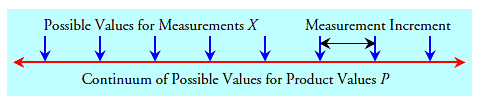Figure 1: Round-off uncertainty

Now the product value (P) does not suffer from the constraint that it has to be some multiple of MI. It can take on any value in the continuum along the line in figure 1. So, when we make a measurement, our observed value will err by some amount, and the difference between X and P will be the round-off error of that measurement, E.

All of the product values P that fall between [X – 0.5 MI] and [X + 0.5 MI] will be represented by the value X. If we assume that these product values are all equally likely, then we have a uniform distribution for those product values that will be represented by the observed value X. With this uniform distribution half of the product values will fall in the interval: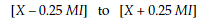This means that half the time the round-off error of our observed value will be less than 0.25 MI (and half the time the error of our observed value will be greater than 0.25 MI).Figure 2: The error of a measurement due to round-off

Thus, 0.25 MI is the median round-off error of a measurement. The relationship between measurement increments and the median round-off error is shown in figure 3. Here the use of smaller measurement increments will result in smaller median errors for our observed values, and using more digits is always good. When round-off is the dominant source of uncertainty in a measurement you will always want to use as many digits as possible when recording the observed values. But round-off is not always dominant.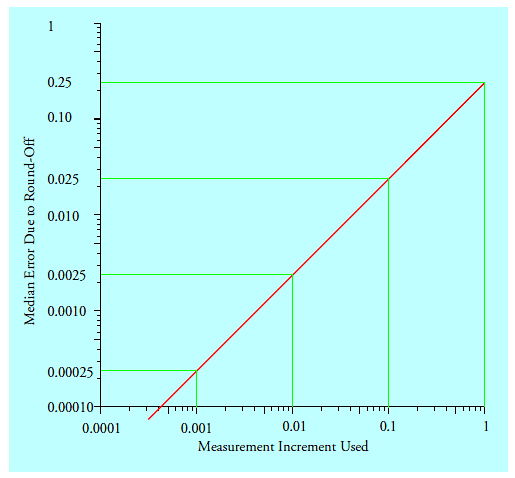Figure 3: The median error due to round-off

### Measurement system error

As we use smaller and smaller measurement increments the uncertainty in the measurement system itself will eventually become the dominant source of measurement error. As before, we consider a product measurement, X, to be the sum of two parts: the product value, P, and the measurement error, E. Further let us assume that the value for E is independent of the value for P. Ever since 1810, when Laplace published what we call the central limit theorem, the standard probability model for the measurement errors E has been a normal distribution. This means that if we measured the same thing thousands of times, using very small measurement increments, we would end up with a histogram like figure 4.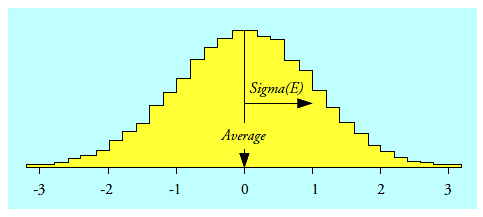Figure 4: Repeated measurements of the same thing do not yield identical values

Here the best estimate of the value for the thing being measured would be the average of all the readings. The estimated standard deviation of these repeated measurements, Sigma(E), would characterize the uncertainty due to the measurement system. Now the normal distribution has the property that the middle 50 percent is defined by the mean plus or minus 0.675 times the standard deviation. Since the early 19th century the name given to 0.675 Sigma(E) has been the probable error (PE):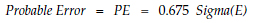Figure 5: The median error of a measurement due to measurement uncertainty

Half the time the observed value X will fall within the central portion of figure 5, and half the time it will fall in one of the tails of figure 5. Thus, the probable error (PE) defines the median error of a measurement due to uncertainty in the measurement system. Regardless of the size of measurement increment used, a measurement will err by one probable error or more at least half the time. If you are going to err by this amount or more half the time, it does not pay to interpret a number more precisely than plus or minus one probable error. Thus, the probable error defines an effective lower bound on the resolution of a measurement, and it does this regardless of how small we make the measurement increment.

While measurement error is commonly reported using the estimated standard deviation, Sigma(E), of the measurement system, we prefer to use the probable error (PE). We do this because it is much easier to get people to understand that “the probable error is the median error introduced by the measurement system” than it is to get them to understand that “Sigma(E) is the radius of gyration for figure 5.” Moreover, since the PE is a simple multiple of Sigma(E), they both contain the same essential information.

### Combining the two sources of uncertainty

If we divide MI by PE we can express the measurement increment as a multiple of the probable error. These values will define the horizontal scale for figure 7. If we divide the median error by PE we express the median error as a multiple of the probable error. These values will define the vertical scale for figure 7. The median error due to round-off alone will be always be one-quarter of the size of the measurement increment, and this relationship creates the sloping line in figure 7. The median error due to measurement uncertainty alone will be equal to the probable error. Since this is independent of the measurement increment used, it is shown in figure 7 as a horizontal line.

When we combine both sources of uncertainty we end up with the median error curve that is tabled in figure 6 and shown in figure 7.Figure 6: Measurement increments and corresponding median errors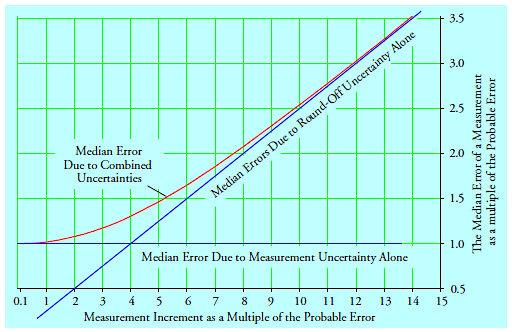Figure 7: The median error of a measurement

On the left, where measurement error is the dominant source of uncertainty, the median error curve is nearly horizontal. As the measurement increment increases round-off begins to affect the measurements and the uncertainty increases. On the right, as round-off becomes the dominant source of uncertainty, the median error curve becomes asymptotic to the sloping line.

The median error curve in figure 7 was independently obtained by each author using simulation studies, and was later verified by direct computation.

### The uncertainty in the last digit

But how does all this stack up against the traditional way of characterizing the quality of a measurement—the uncertainty in the last digit? To answer this question consider what hap­pens when, for example, a measurement has an increment equal to 2.2 PE units. According to the table in figure 6, when the measurement increment is 2.2 PE units, the median error of a measurement will be 1.094 times the probable error.

When we divide [1.094 PE units] by [2.2 PE units per measurement increment] we find that the median error of these measurements will be equal to one-half measurement increment: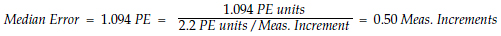Thus, when the measurement increment equals 2.2 PE units, the measurements will err by one-half of a measurement increment or less at least half the time. Such measurements will be “good to the last increment” over half the time.

Inspection of the formula above will show that in order to express the median error in measurement increments we simply divide a value from the second column of figure 6 by the corresponding value in the first column. The results of this exercise are summarized in figure 8. Figure 9 shows the third column of figure 8 as a function of the first column of figure 8.Figure 8: Median errors expressed in measurement increments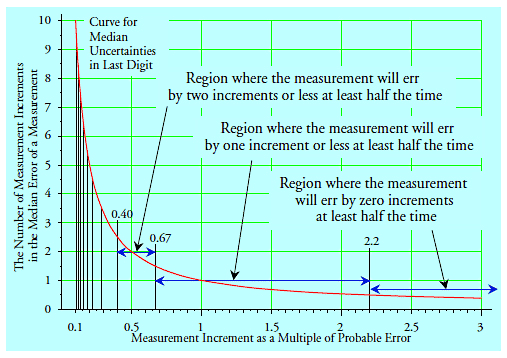Figure 9: The median uncertainty in the last digit

For the usual case where the measurement increment is some power of 10, the curve in figure 9 and the values in figure 8 define the uncertainty in the last digit. Specifically, whenever a measurement increment exceeds 2.2 probable errors it will err by zero digits in the last place at least half the time.

Measurement increments that fall between 0.67 PE units and 2.2 PE units will err by one or fewer digits in the last place at least half the time. Such values might be said to be good to within one digit in the last place.

Measurement increments that fall between 0.40 PE units and 0.67 PE units will err by two or fewer digits in the last place at least half the time. Such values might be said to be good to within two digits in the last place.

Measurement increments that fall between 0.28 PE units and 0.40 PE units will err by three or fewer digits in the last place at least half the time. Such values might be said to be good to within three digits in the last place.

Measurement increments that fall between 0.22 PE units and 0.28 PE units will err by four or fewer digits in the last place at least half the time. Such values might be said to be good to within four digits in the last place.

As the measurement increment drops from 0.22 PE units down to 0.10 PE units the median error rapidly climbs from five increments to 10 increments.

Measurement increments that are powers of ten which fall below 0.10 PE units have no useful information in the last recorded digit, since they will err by more than 10 digits in the last place at least half the time.

### The twin uncertainties affecting every measurement

In figures 7 and 9 we have the twin uncertainties that affect all measurements. Since both graphs have the same horizontal axis we combine the two curves to get figure 10. There the vertical scale on the left shows the number of measurement increments that correspond to the median error of a measurement while the scale on the right shows the actual median error in PE units.Figure 10: The twin uncertainties for every measurement

Figure 10 shows the twin uncertainties that affect every measurement. Large measurement increments will have less uncertainty in the last digit, but they will have unnecessarily large median errors. Very small measurement increments will have the minimum median error, but they will be exceedingly soft in the last digit. Thus the choice of a measurement increment requires a trade-off.

When the measurement increment falls between 0.67 PE units and 1.0 PE unit the measurements will be good to within one digit in the last place and they will have a median error that is within 2 percent of the minimum. This is the zone marked as Best in figure 10.

The zone marked Good in figure 10 extends from measurement increments of 0.40 PE units up to 1.5 PE units. On the left side of this zone the measurements will be good to within two digits in the last place and will have a median error of less than 1.009 PE. On the right side they will be good to within one digit in the last place and will have a median error below 1.044 PE.

The zone marked Acceptable in figure 10 extends from measurement increments of 0.22 PE units up to 2.2 PE units. On the left side the measurements will be good to within four digits in the last place and will have a median error of less than 1.003 PE. On the right side they will be good to within one digit in the last place and will have a median error below 1.094 PE. Since this zone gives us an order of magnitude to play with, it effectively defines the range of appropriately sized measurement increments to use.

Finally, since the error of recording too many digits is less severe than that of recording too few digits, we could classify measurements with increments in the range of 0.10 PE unit to 0.22 PE units as very soft but usable. (These measurements will err by five units or more in the last place at least half the time, but will have a median error of 1.001 PE or less).

### Using the right number of digits

When we combine all of the information above we end up with the guidelines shown in figure 11. There the horizontal scales show the ranges for the size of the measurement increment in terms of both the probable error and the standard deviation of measurement error, Sigma(E). Depending on where your measurement increment falls, the diagram describes the dominant uncertainty in your measurements and the appropriate course of action you should take.Figure 11: Using the right number of digits

### Large measurement increments

Since the median error will increase rapidly when the measurement increment exceeds 2.2 PE units and since recording one additional digit will convert such a measurement into one that has a measurement increment that is in the acceptable zone, you should consider adding a digit to your recorded values whenever the measurement increment exceeds 2.2 PE units.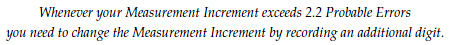### Example one

Say, for example that you have measurements recorded to the hundredth of a millimeter and that the probable error of the measurement system is PE = 0.0014 mm. Then, when we divide the measurement increment by the probable error we discover that the measurement increment is equal to 0.01/0.0014 = 7.1 probable errors and we are in the region where round-off is the dominant source of uncertainty.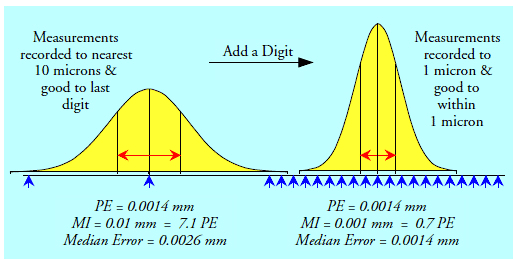Figure 12: Example one

From figure 6 we can compute the median error of our measurements to be approximately 1.85 PE = 0.0026 mm. From figure 9 we see that with a measurement increment of 7 PE our measurements will err by zero digits in the last place at least half the time. Thus, these measurements will be good to the nearest hundredth of a millimeter, but they are not as good as the measurement system will allow them to be.

If it was economically feasible to add one extra digit to these measurements, so that the measurement increment would become 0.001 mm, then the measurement increment would be equal to 0.71 probable errors. From figure 6 the median error of a measurement would be about 1.010 PE = 0.0014 mm. From figure 9 these measurements would be good to within one digit in the last place at least half the time. Recording one extra digit here reduces the median error from 0.0026 mm to 0.0014 mm, effectively cutting the uncertainty in the measurements in half.

### Small measurement increments

When the measurement increment is less than 0.10 PE units the measurements will err by 10 digits or more in the last place at least half the time. Clearly this is a zone where we should drop the last recorded digit. By doing so we will not appreciably change the precision of the measurements.

“But I prefer to write more digits!”

Writing down random numbers does not make the measurements “more precise.” It just makes them look better than they actually are. When we insist on using a measurement increment that is smaller than 0.10 probable errors we are misrepresenting the precision of the measurements to ourselves and others. Just because some extra digits show up on a digital read-out does not make the extra digits real.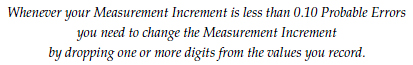### Example two

Say, for example that you have a scale that records weight to the nearest microgram (MI = 1 microgram). However, let us assume that repeated measurements of a standard weight show that the standard deviation of the measurement system is Sigma(E) = 17 micrograms. Then the probable error for these measurements is PE = 11.5 micrograms. This means that while you may be recording weights to the nearest microgram, they are going to err by 12 micrograms or more at least half the time, and your last recorded digit will be nothing but noise.

When we divide the measurement increment by the probable error we discover that the measurement increment is equal to 1/11.5 = 0.087 PE. Since this is less than 0.10 PE we are in the left hand box of figure 11, and we need to drop a digit from our recorded values.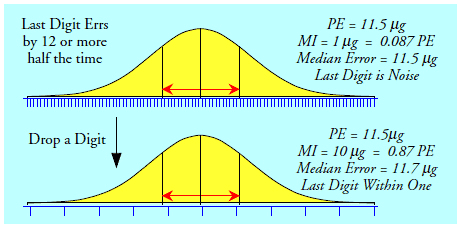Figure 13: Example two

By rounding our weights to the nearest 10 micrograms our measurement increment becomes MI = 10 micrograms or 0.87 PE. Interpolating in figure 6 we find that our median error will increase slightly to 1.017 PE = 11.7 micrograms, and our recorded values will be good to within one in the last place at least half the time. Rounding these values to the nearest multiple of 10 micrograms prevents these measurements from looking better than they really are, makes the values good to within one digit in the last place, and does not appreciably increase the uncertainty in the measurements.

### Soft and very soft measurements

Very soft measurements have an increment between 0.10 PE and 0.22 PE and will have a median error of 5 to 10 in the last digit at least half the time. While these measurements may occasionally be of use, our recommendation is to make the measurement increment five or 10 times larger. This will reduce the median uncertainty to one or two measurement increments without an undue increase in the median error of a measurement.

Soft measurements have an increment between 0.22 PE and 0.40 PE and will err by 3 to 4 in the last digit at least half the time. While these soft measurements may be used in this form, doubling the size of the measurement increment will reduce the median uncertainty to one or two increments without an undue increase in the median error of a measurement.

### Summary

Using a measurement increment that is too large will throw away useful information. Using a measurement increment that is too small will add noise to your measurements.

With the information provided here you no longer need to guess about how many digits you need to record. You can now determine exactly how many digits are appropriate for any measurement system. Once you have an estimate for the standard deviation for the measurement system you can easily obtain the probable error. Once you know the probable error you know the effective resolution of the measurements. You can then make your own trade-offs to determine how many digits to record, Acceptable measurement increments will fall between 0.22 probable errors and 2.2 probable errors. (In practice these cut-offs may be rounded to 0.2 PE and 2 PE.)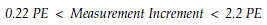Moreover, since you can determine these values for your own measurement system, operated by your own personnel, in your plant, you no longer have to depend upon the assurances of some salesman about the precision of a measurement system. Neither are tradition and guesswork needed. Now you have a way to know how good your measurements are, and how many digits to record.

### Discuss### Donald J. Wheeler

Dr. Wheeler is a fellow of both the American Statistical Association and the American Society for Quality who has taught more than 1,000 seminars in 17 countries on six continents. He welcomes your questions; you can contact him at djwheeler@spcpress.com.### James Beagle III

James Beagle III is a lapsed CQE, CRE, and C6SBB (asking the wrong questions and obsessing about impractical concepts, before realizing what is important) with 35+years experience in process, quality, and reliability engineering. He primarily works with a large manufacturing base supplying data storage component products, where he develops tools for data analysis, designs experiments for product improvements, and works on product and process qualification. In his free time he works on numerical modeling (and is also known to have guitars at home). He can be reached at james@idealstates.net.